# Free online coursePrealgebra by Leonard

3.46

(48)

## 38h54m of online video course

Quality content and selected for your learning.

## Certificate

Download your digital certificate of course completion after watching all course videos.

## Exercises to train your knowledge

Many of the courses available have exercises to help with learning.

## Free lifetime access

This course is free today and always will be, from start to finish you will pay nothing.

## Algebra

Study for free algebra, linear algebra, pre algebra and other with the free courses offered here. Our courses are the best free in all the webTeacher

##### Leonard
###### 6 Courses / 1743 students

This Channel is dedicated to quality mathematics education. It is absolutely FREE so Enjoy! Videos are organized in playlists and are course specific.

## Course content preview

There are no comments yet for this course.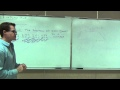Watch the 1st video of the course

## Course content0h14m

### Prealgebra Lecture 1.2: Studying Place Value and Expanded Form of Numbers

Prealgebra Lecture 1.2: Studying Place Value and Expanded Form of Numbers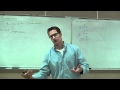0h44m

### Prealgebra Lecture 1.3: Addition and Subtraction of whole numbers. Perimeter.

Prealgebra Lecture 1.3: Addition and Subtraction of whole numbers. Perimeter.0h23m

### Prealgebra Lecture 1.4: How to do Rounding and Estimating of Whole Numbers

Prealgebra Lecture 1.4: How to do Rounding and Estimating of Whole Numbers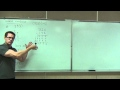0h45m

### Prealgebra Lecture 1.5: Multiplication of Whole Numbers. Area of a Rectangle

Prealgebra Lecture 1.5: Multiplication of Whole Numbers. Area of a Rectangle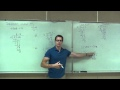0h46m

### Prealgebra Lecture 1.6: Division of Whole Numbers

Prealgebra Lecture 1.6: Division of Whole Numbers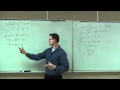1h15m

### Prealgebra Lecture 1.7: Studying Exponents and Order of Operations

Prealgebra Lecture 1.7: Studying Exponents and Order of Operations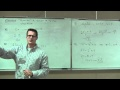1h01m

### Prealgebra Lecture 1.8: An Introduction to Variables and Algebraic Expressions

Prealgebra Lecture 1.8: An Introduction to Variables and Algebraic Expressions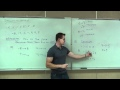0h38m

### Prealgebra Lecture 2.1: Introduction to Integers

Prealgebra Lecture 2.1: Introduction to Integers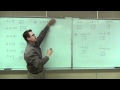0h37m

### Prealgebra Lecture 2.2: Adding of Integers. Using the Addition Rule for Integers

Prealgebra Lecture 2.2: Adding of Integers. Using the Addition Rule for Integers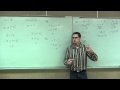0h56m

### Prealgebra Lecture 2.3: Subtracting Integers. How to Change Subtraction to Addition

Prealgebra Lecture 2.3: Subtracting Integers. How to Change Subtraction to Addition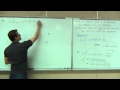0h50m

### Prealgebra Lecture 2.4: Multiplying and Dividing Integers

Prealgebra Lecture 2.4: Multiplying and Dividing Integers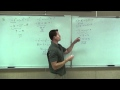2h00m

### Prealgebra Lecture 2.5: Studying Order of Operations with Integers

Prealgebra Lecture 2.5: Studying Order of Operations with Integers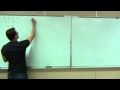1h22m

### Prealgebra Lecture 2.6: An Introduction to Solving Basic Equations

Prealgebra Lecture 2.6: An Introduction to Solving Basic Equations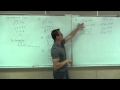2h17m

### Prealgebra Lecture 3.1: Simplifying Algebraic Expressions

Prealgebra Lecture 3.1: Simplifying Algebraic Expressions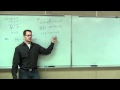1h23m

### Prealgebra Lecture 3.2: Solving Basic Equations

Prealgebra Lecture 3.2: Solving Basic Equations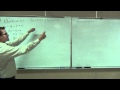1h14m

### Prealgebra Lecture 3.3: Solving General Equations. How to Solve Any Equation in Prealgebra

Prealgebra Lecture 3.3: Solving General Equations. How to Solve Any Equation in Prealgebra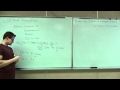0h28m

### Prealgebra Lecture 3.4: Translating Sentences Into Equations. Intro to Word Problems

Prealgebra Lecture 3.4: Translating Sentences Into Equations. Intro to Word Problems0h30m

### Prealgebra Lecture 4.1: Introduction to Fractions

Prealgebra Lecture 4.1: Introduction to Fractions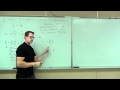1h16m

### Prealgebra Lecture 4.2: Prime Factorization and Simplification of Fractions

Prealgebra Lecture 4.2: Prime Factorization and Simplification of Fractions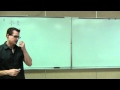1h53m

### Prealgebra Lecture 4.3: How to Multiply and Divide Fractions

Prealgebra Lecture 4.3: Multiplying and Dividing Fractions